Relative vs. Absolute Cell References in Spreadsheets

In working with spreadsheets, you need to know about relative vs. absolute cell references.

Here is the issue: when you COPY A FORMULA that contains cell references, what happens to the cell references?

Usually the CELL REFERENCES will CHANGE! If you copy a formula 2 rows to the right, then the cell references in the formula will shift 2 cells to the right. If you copy a formula 3 rows down and 1 row left, then the cell references in the formula will shift 3 rows down and 1 row left. These are called "relative" cell references, since they change relative to where you copy the formula.

If you do not want cell references to change when you copy a formula, then make those cell references absolute cell references. Place a "\$" before the column letter if you want that to always stay the same. Place a "\$" before a row number if you want that to always stay the same. For example, "\$C\$3" refers to cell C3, and "\$C\$3" will work exactly the same as "C3", expect when you copy the formula. Note: when entering formulas you can use the F4 key right after entering a cell reference to toggle among the different relative/absolute versions of that cell address.

The trick in creating spreadsheets is deciding before you copy a formula what cell references in the formula you want to be relative and what you want to be absolute. If some cell references refer to input cells in the spreadsheet, you usually want those cells to be absolute.

The article below gives further instruction in absolute vs. relative cell references.

Relative & Absolute Cell Referencesby Karyn Stille

Excel uses two types of cell references to create formulas.  Each has its own purpose.  Read on to determine which type of cell reference to use for your formula.

Relative Cell References

This is the most widely used type of cell reference in formulas.  Relative cell references are basic cell references that adjust and change when copied or when using AutoFill.

Example:

=SUM(B5:B8), as shown below, changes to =SUM(C5:C8) when copied across to the next cell.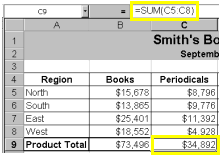Absolute Cell References

Situations arise in which the cell reference must remain the same when copied or when using AutoFill.  Dollar signs are used to hold a column and/or row reference constant.

Example:

In the example below, when calculating commissions for sales staff, you would not want cell B10 to change when copying the formula down.  You want both the column and the row to remain the same to refer to that exact cell.  By using \$B\$10 in the formula, neither changes when copied.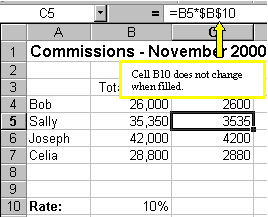A more complicated example:

Let's pretend that you need to calculate the prices of items in stock with two different price discounts. Take a look at the worksheet below.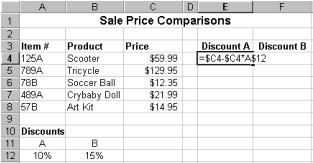Examine the formula in cell E4. By making the first cell reference \$C4, you keep the column from changing when copied across, but allow the row to change when copying down to accommodate the prices of the different items going down.  By making the last cell reference A\$12, you keep the row number from changing when copied down, but allow the column to change and reflect discount B when copied across.  Confused?  Check out the graphics below and the cell results.

Copied Across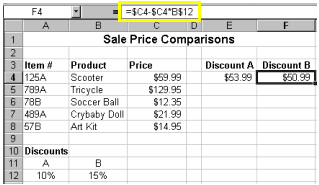Copied Down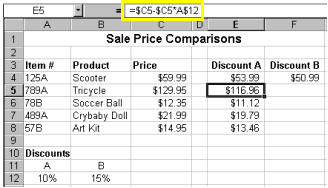Now, you might be thinking, why not just use 10% and 15% in the actual formulas?  Wouldn't that be easier? Yes, if you are sure the discount percentages will never change - which is highly unlikely.  It's more likely that eventually those percentages will need to be adjusted.  By referencing the cells containing 10% and 15% and not the actual numbers, when the percentage changes all you need to do is change the percentage one time in cell A12 and/or B12 instead of rebuilding all of your formulas. Excel would automatically update the discount prices to reflect your discount percentage change.

Summary of absolute cell reference uses:

 \$A1 Allows the row reference to change, but not the column reference. A\$1 Allows the column reference to change, but not the row reference. \$A\$1 Allows neither the column nor the row reference to change.

There is a shortcut for placing absolute cell references in your formulas!

When you are typing your formula, after you type a cell reference - press the F4 key.  Excel automatically makes the cell reference absolute!  By continuing to press F4, Excel will cycle through all of the absolute reference possibilities.  For example, in the first absolute cell reference formula in this tutorial, =B4*\$B\$10, I could have typed, =B4*B10, then pressed the F4 key to change B10 to \$B\$10.  Continuing to press F4 would have resulted in B\$10, then \$B10, and finally B10. Pressing F4 changes only the cell reference directly to the left of your insertion point.

I hope this tutorial has made these cell reference types "absolutely" clear!

Microsoft Office Tutorials
http://tutorials.esmartweb.com